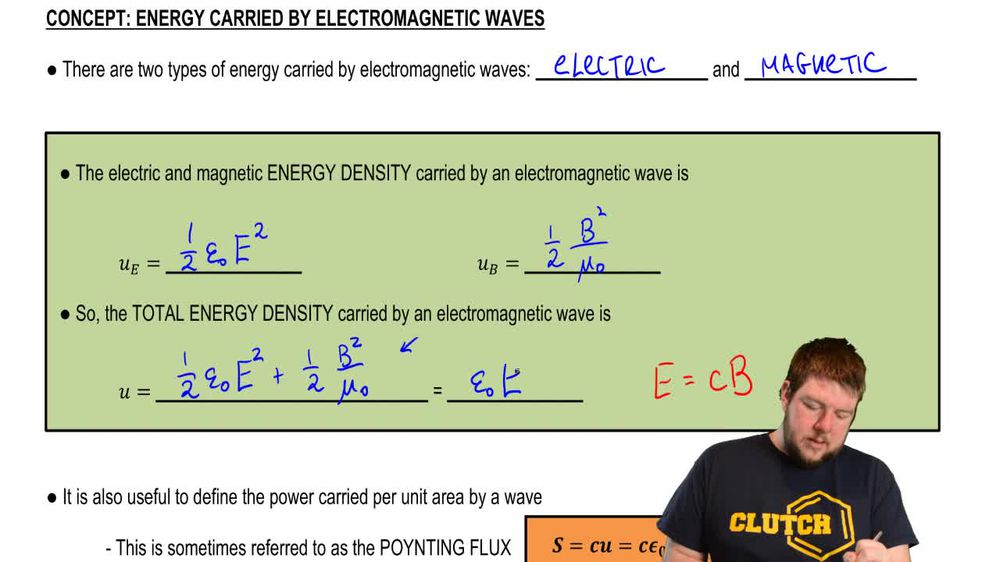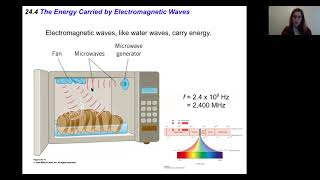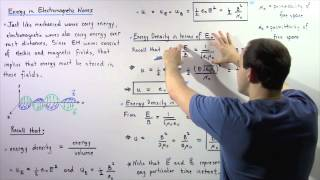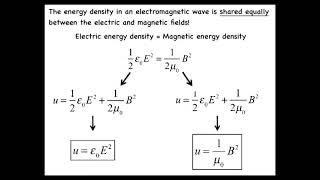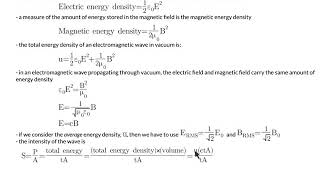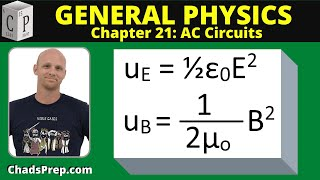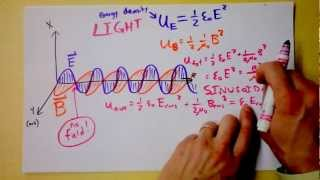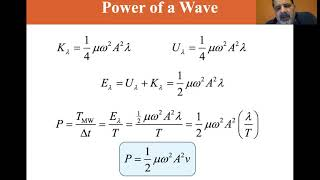Start typing, then use the up and down arrows to select an option from the list.
1. 32. Electromagnetic Waves2. Energy Carried by Electromagnetic Waves# Energy Carried by Electromagnetic Waves

by Patrick Ford
143 views
1
2
1
Hey, guys. In this video, we're gonna talk about the energy carried by electromagnetic waves and a quantity related to the energy called the intensity. Okay, let's get to it. Now. There are two types of energy carried by electromagnetic waves. As the name would imply there is electric energy and there is magnetic energy. Now, the way the energy is typically discussed in the context of electromagnetic waves is always by discussing the energy density. Remember, little you lower case you. The energy density is the energy per unit volume. Okay, the energy density carried by an electromagnetic wave is split up into those two categories. You have electric energy, which is one half absolutely not E squared. Okay, whatever the electric field strength happens to be and the magnetic energy, which is one half b squared over mu, not whatever the magnetic field strength happens to be right. Obviously. Mawr, magnetic field strength, More electric field strength, Mawr energy. Okay, So the total carry the total energy density carried by an electromagnetic wave is just the some of those two parts of energy that it carries one half absolutely not e squared, plus one half B squared over mu. Not now. The thing to remember is that the electric field strength in the magnetic field strength are always related to one another in an electromagnetic wave. Okay, we've seen instances before where we talked about individual charges producing an electric field or a moving charge producing a magnetic field or a moving charge in the magnetic field in an electric field, etcetera. Those were disconnected electric and magnetic fields. When you're talking specifically about an electromagnetic wave where you have paired electric and magnetic fields producing light, then the electric field strength the magnetic field strength are always related to each other by the speed of light. Okay? And if you use the substitution for what speed of light is the definition of the speed of light which we saw before, which depends upon the permitted ity and the permeability, you can rewrite this summation as simply absolutely not e squared. Okay, this is the simplest way to represent it. Just know what the electric field strength is of your electromagnetic wave. Square it. Multiply it by the permitted ity of free space By absolutely not. Okay. Now it's useful to define a quantity that is known as the pointing flux, which is the power carried per unit area by a wave, Okay, by an electromagnetic wave. Now, the thing is that because electromagnetic waves oscillate the pointing flux oscillates as well. Remember that in an electromagnetic wave, minimize myself. You're gonna have an electric field that's all slipping up and down. Right, So here you have no electric field, so you have no pointing flux. Here. You have a maximum electric field, so you have a maximum pointing flux here. You have no electric field, minimum pointing flux. Here you have maximum electric field again, it's negative. But the pointing flux depends upon the electric field squared. So you have the maximum pointing flux again. Okay, Now the intensity of the wave, which is the average of the pointing flux, that is a very, very important thing to know. Okay, that tells you, on average how much power is delivered or how much power is carried by a wave per unit area on average. Okay. And with these Sinus soil waves, the average of e squared. OK, this is something that we talked about, or something that you saw during alternating currents, that the average of e itself is gonna be zero, but the average of the squared is gonna be one half he squared. OK, so that's where you get this one half from. So that intensity is just one half times. Absolutely not. Which is the permitted of free space times. See, which is the speed of light times E squared Max. Now, I specifically put maximum indicating these positions. Right. That is the amplitude of the oscillation. Alright, guys, let's do a quick example and then be done with this. A laser outputs light at an average of 0.5 Nana watts, if the light is emitted from the laser and it's cylindrical beam of radius 0.5 millimeters, what's the intensity of the light? What is the maximum value of the electric field? Okay, the intensity is just gonna be the average power per unit area. In this case, the beam is emitted cylindrical E. So the area is just gonna be that front circular cross section of the cylinder. Okay, so the area is just gonna be that area of the cylinder and its radius this 0.5 millimeters. All right. The intensity is just gonna be that average power divided by that area. The average power, we're told his five Nana Watts Nano is 10 to the negative nine. The area of a circle is just pi r squared, right? So pi times 0.5 millimeters millions 10 to the negative three. Don't forget squared. And that whole thing equals 0. watts per meter squared right. Powers in units of watts and area is in the units of meters squared. Okay, Now, if we want to find the maximum electric field, which is the next part of the question, we simply need to use this equation. We can also say that the intensity is one half absolutely not. See times e max squared. I wanna multiply the two up divide both the absolutely not and the sea over. And then I need to take the square root. Okay, So e max is the square root of two times I write. I multiplied the two up. I divided Absalon not and see. This whole thing is two times 20. Divided by 8.85 times 10 to the negative. 12 three times 10 to the eighth. If you don't remember those numbers. That's fine. You can just look them up. You're not expected to really remember them. The speed of light you will be expected to remember, But not absolutely not. Right? This whole thing is just 069 Newtons per cool. Um Alright, guys, that wraps up our discussion on the energy carried by these electromagnetic waves. Thanks for watching.